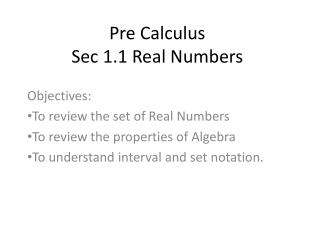DownloadDownload PresentationPre Calculus Sec 1.1 Real Numbers

# Pre Calculus Sec 1.1 Real Numbers

Télécharger la présentation## Pre Calculus Sec 1.1 Real Numbers

- - - - - - - - - - - - - - - - - - - - - - - - - - - E N D - - - - - - - - - - - - - - - - - - - - - - - - - - -
##### Presentation Transcript

1. Pre CalculusSec 1.1 Real Numbers Objectives: To review the set of Real Numbers To review the properties of Algebra To understand interval and set notation.

2. Real Numbers • Natural Numbers: 1,2,3,4,… • Integers: -,…-3,-2,-1,0,1,2,3,… • Rational Numbers: any # that can be written as a ratio of integers (as a fraction). • Irrational Numbers: any # that cannot be written as a fraction.

3. CLASS WORK • Given the set, list the elements of the set that are: • Natural numbers • Integers • Rational numbers • Irrational numbers

4. Properties of Real Numbers Commutative Property: a + b = b + a ab = ba order doesn’t matter Associative Property: (a+b)+c = a+(b+c) (ab)c = a(bc) order doesn’t change

5. Distributive Property: a(b+c) = ab + ac you can add then multiply or multiply then add.

6. CLASS WORK State the property of real numbers being used. 2. 3. 4.

7. Sets & Elements • A set is a collection of objects. - the objects are called the elements of the set. If S is a set, the notation of means that a is an element of S.

8. Sets & Elements means that b is not an element of S. Ex1. If Z represents the set of integers, then but

9. Notation of Sets • Braces { } - The set A that consists of positive integers less than 7 is • Set-builder notation – • Interval notation – These are sets of real numbers and correspond geometrically to line segments.

10. Union of Sets • If S and T are sets, then , represents their union. The union of sets consists of all elements in both sets. Ex 2. Find if

11. Intersection of Sets • The intersection of S and T is the set consisting of all elements that are in both sets. It is only what they have in common. Ex 3. Find if

12. Ex 4. Find, , ,

13. CLASS WORK If find: 5. 6. 7.

14. CLASS WORK If find 8. 9. 10.

15. Intervals b b

16. Ex. 5 Express each interval in terms of inequalities then graph the interval. • [-1, 2) • [1.5, 4] • (-3, )

17. CLASS WORK Express each interval in terms of inequalities then graph the interval. 11. [2, 8) • (-, -5)

18. CLASS WORK Express the inequality in interval notation, then graph the interval. 13. 14.

19. HW p. 10 1-9 odd, 33,34,35-59 odd